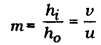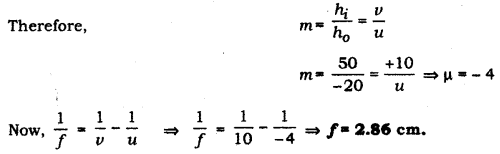# An object 50 cm tall is placed on the principal axis of a convex lens. Its 20 cm tall image

An object 50 cm tall is placed on the principal axis of a convex lens. Its 20 cm tall image is formed on the screen placed at a distance of 10 cm from the lens. Calculate the focal length of the lens.

Object distance, image distance, object height and image height are related as:Where, m is the magnification of the image.
It is given that:
Image distance (v) = + 10 cm.
Height of object (h0) = 50 cm
Height of image (h1) = - 20 cm
(It is negative because a real image formed by a convex lens is always inverted)